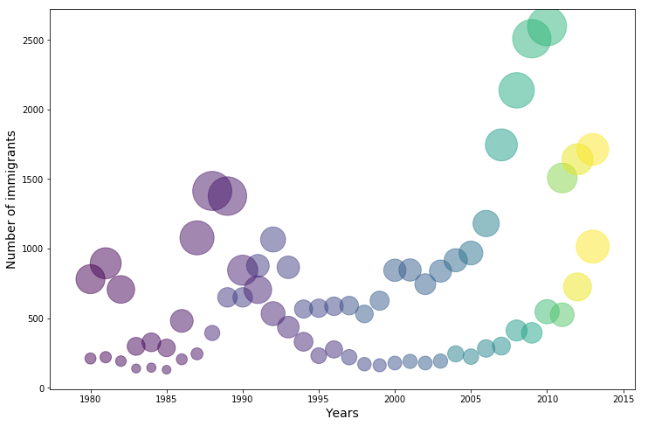1595667480

# Bubble Plots in Matplotlib

Bubble plots are an improved version of the scatter plot. In a scatter plot, there are two dimensions x, and y. In a bubble plot, there are three dimensions x, y, and z. Where the third dimension z denotes weight. That way, bubble plots give more information visually than a two dimensional scatter plot.

## Data Preparation

For this tutorial, I will use the dataset that contains Canadian immigration information. It has the data from 1980 to 2013 and it includes the number of immigrants from 195 countries. import the necessary packages and the dataset:

``````import numpy as np
import pandas as pd
skiprows=range(20),
skipfooter=2)
``````

The dataset is too big. So, I can not show a screenshot here. Let’s see the name of the columns.

``````df.columns

#Output:
Index([    'Type', 'Coverage',   'OdName',     'AREA', 'AreaName',      'REG',
'RegName',      'DEV',  'DevName',       1980,       1981,       1982,
1983,       1984,       1985,       1986,       1987,       1988,
1989,       1990,       1991,       1992,       1993,       1994,
1995,       1996,       1997,       1998,       1999,       2000,
2001,       2002,       2003,       2004,       2005,       2006,
2007,       2008,       2009,       2010,       2011,       2012,
2013],
dtype='object')
``````

We are not going to use a lot of the columns. I just dropped those columns and set the name of the countries (‘OdName’) as the index.

``````df = df.drop(columns = ['Type', 'Coverage', 'AREA', 'AreaName',      'REG', 'RegName', 'DEV', 'DevName',]).set_index('OdName')
``````

I chose the data of Ireland and Brazil for this exercise. There is no special reason. I chose them randomly.

``````Ireland = df.loc['Ireland']
Brazil = df.loc['Brazil']
``````

## Normalize the Data

There are a few different ways to normalize the data. We normalize the data to bring the data in a similar range. Ireland and Brazil immigration data have different ranges. I needed to bring them to the range from 0 to 1. I simply divided the Ireland data by the maximum value of the Ireland data series. I did the same to the data Series of Brazil.

``````i_normal = Ireland / Ireland.max()
b_normal = Brazil / Brazil.max()
``````

We will plot the Ireland and Bazil data against the years. It will be useful to have the years on a list.

``````years = list(range(1980, 2014))
``````

## Make the Bubble Plot

Just to see the difference, let’s plot the scatter plot first.

``````import matplotlib.pyplot as plt
plt.figure(figsize=(14, 8))
plt.scatter(years, Ireland, color='blue')
plt.scatter(years, Brazil, color='orange')
plt.xlabel("Years", size=14)
plt.ylabel("Number of immigrants", size=14)
plt.show()
``````

Now, plot the bubble plot. We have to input the size that we defined before.

#python #data-visualization #data-science #matplotlib #data analysis

## Buddha Community1584424980

## Matplotlib Tutorial - Bar Charts and reading in CSV Data - (Part 2)

Matplotlib Tutorial - Bar Charts and reading in CSV Data - (Part 2)
Part 2 of our Matplotlib Tutorial Videos Series is out…

In this video, we will learn creating bar charts in matplotlib. We will also learn to put bar charts side-by-side in matplotlib. Also, we will read in a csv file to create bar charts in Matplotlib .

#matplotlib #barcharts #pythonplotting #matplotlibtutorials #matplotlibvideos

#matplotlib #Matplotlib-tutorial-videos #matplotlib-plotting #matplotlib-bar-charts1621948980

## The Matplotlib Line Plot that Crushes the Box Plot

Intuitively show statistics, error bars, or custom functions

The box plot is a quick and convenient way to get a feel for your data set — it can give you a snapshot of your range, mean, quartiles, and outliers. Sure, it’s not as descriptive as a histogram or kde for the distribution, but it’s fantastic for seeing how our distributions change over our variables.

While data scientists and most technical people are familiar and comfortable with box plots, they can be pretty foreign to people in non-engineering or statistics domains. And herein lies the problem: you need a way to show how your data trends and its distributions change in a format that anyone can understand.

I’m going to show you step-by-step how to make a line plot that conveys as much or as little information from the box plot that you’d like to share — means, medians, ranges, quartiles, standard deviation error bars, or any custom values.

#box-plot #pandas #line-plot #visualization #matplotlib1592385414

## Python Matplotlib Plotting BoxPlot

This is our seventh article in Python Matplotlib, in this article we are going to learn about Matplotlib Plotting BoxPlot. so in descriptive statistics, a box plot or boxplot is a method for graphically depicting groups of numerical data through their quartiles. Box plots may also have lines extending from the boxes indicating variability outside the upper and lower quartiles, hence the terms box-and-whisker plot and box-and-whisker diagram. also Boxplot allows you to compare distributions of values by conveniently showing the median, quartiles, maximum, and minimum of a set of values.

#matplotlib #python tutorials #matplotlib boxplot #python matplotlib1592313180

## Python Matplotlib Plotting Barchart - Code Loop

This is our fourth article in Python Matplotlib, in this article we are going to learn about Matplotlib Plotting Barchart.

#matplotlib #matplotlib barchart #python #python matplotlib1625815800

## Scatter Plots - Python Matplotlib Tutorial #3

In this Python tutorial, you will learn everything you need to know about scatter plots using Matplotlib library. Scatter plots are great in showing the relationship between variables in our dataset, eventually revealing a correlation.

This is a complete tutorial on scatter plots including Bubble plots and an additional variable mapped to the marker’s color (you will also learn how to add colorbar and choose the best colormap to your case). We will discuss how to change the marker of your scatter plot using two different methods: 1. Symbols from Matplotlib and 2. Symbols from Latex. As usual, I will share several tips and tricks to save your time when customizing your graph.

We will cover from the very basic to more advanced aspects in 15 minutes. I hope you enjoy this tutorial.

Playlist: Matplotlib Course | Video #3